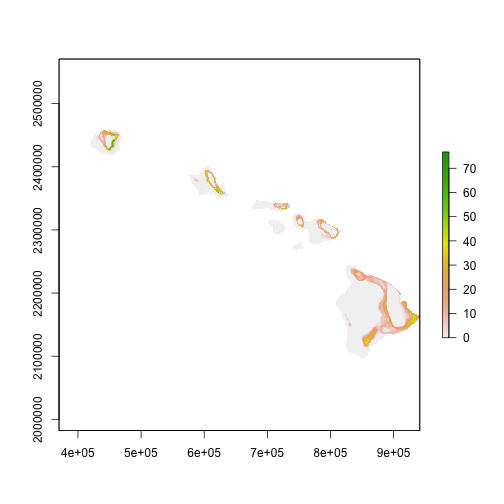# HICROP in R

HICROP input variables include topographic, edaphic, and climatic variables to identify spatial niche suitability for selected crops.

The topographic and edaphic variables are: slope, soil depth, soil drainage, and soil pH.

First, make some basic functions

``````library(raster)
library(dplyr)

# Local variables:
# function to prompt user to input crop name
return(toString(n))
}

# PROMPTS USER TO INPUT CROP NAME

crop_db <-  read.csv("CROPS.csv", stringsAsFactors = F) #insert csv file path here

# Use col name of variable to index crop_db and return value
locate <- function(var) {
n <- unique(eval(parse(text=(paste0("crop_db\$",var,"[crop_db\$NAME == crop]")))))
return(n)
}

# General suitability function
suit4 <- function(x) { ifelse(x < ABSmin, 0, # Conditional statement for values less than absolute minimum
ifelse(x > ABSmax, 0, # Conditional statement for values greater than absolute maximum
ifelse(((x >= OPTmin) & (x <= OPTmax)),100, # Conditional statement for values greater than or equal absolute maximum AND less than or equal to optimal maximum (i.e. the ideal zone)
ifelse(((x <= ABSmax) & (x > OPTmax)),((1-(x-OPTmax)/(ABSmax-OPTmax))*100), # Conditional statement for values less than or equal absolute maximum AND greater than optimal maximum
ifelse(((x >= ABSmin) & (x < OPTmin)),(x-ABSmin)/(OPTmin-ABSmin)*100,NA ) )))) } # Conditional statement for values greater than or equal absolute minimum AND less than optimal maximum
``````
``````# Call package libraries
library(rgdal)
library(raster)
library(RColorBrewer)

setwd("/Users/hh/Documents/GitHub/HICROP")

# Define Input Variables from TIFs
SLOPE <- raster("~/Documents/GitHub/HICROP/Inputs/soils/Slope_statewide1.tif")
DEPTH <- raster("~/Documents/GitHub/HICROP/Inputs/soils/depth_statewide1.tif")
DRAIN <- raster("~/Documents/GitHub/HICROP/Inputs/soils/drainage_statewide_new1.tif")

# # Take a peek
# #Slope
# plot(SLOPE, col= terrain.colors(5), main="Slope (%)")
# #Depth
# craypas <- brewer.pal(11,"PuOr")
# plot(DEPTH, main='Soil Depth (cm)', col= craypas)
# #Drainage
#
# # PH
# plot(PH, breaks = c(0,1,2,3,4,5,6,7,8,9,10,11,12,13,14), col = rainbow(14), main="Soil pH", ylab = "UTM Northing Coordinate (m)",xaxt='n',yaxt='n', xlab = "UTM Westing Coordinate (in messed up scientific notation)")
#
# # Drainage
# ryb <- brewer.pal(10,"RdYlBu")
# plot(DRAIN, main = "Drainage in HI", breaks = c(0:9), col = ryb, sub = "Float recoded from string in EcoCrop. 1 = subaqueous, 8 = Excessively Drained")
``````

# Going From Python/ArcGIS to R

The goal is to spit out a suitability map for a given model parameter (e.g., slope, soil pH, soil depth etc). A fuzzy math function is used to evaluate suitabiity based on optimal and absolute ranges.

Previous version of the model have run with a set of python scripte and ArcGIS modelbuilder operations. These function well, but take a long time to run. In order to speed things up and be able to programatically perform far greater volumes of analysis the model is being converted into R.

### Ph Suitability

``````PH <- raster("inputs/soils/ph_statewide1.tif")

# get variables
OPTmin <- locate("P_OPTMIN")
OPTmax <- locate("P_OPTMAX")
ABSmin <- locate("P_ABSMIN")
ABSmax <- locate("P_ABSMAX")

print(paste("Optimal soil pH range for", crop,"is:", OPTmin,"-",OPTmax))
``````
```##  "Optimal soil pH range for Banana is: 5 - 7"
```
``````print(paste("Absolute soil pH range for", crop,"is:", ABSmin,"-",ABSmax))
``````
```##  "Absolute soil pH range for Banana is: 4.5 - 7.5"
```
``````# calculate suitability
P_suit <- calc(PH, suit4)

# plot output
plot(P_suit, main= paste("Ph Suitability for ",crop), breaks = c(0,25,50,75,100), col = rainbow(5),
sub=paste("Optimal pH range:", OPTmin,"-",OPTmax, "\n Absolute pH range:", ABSmin,"-",ABSmax))
``````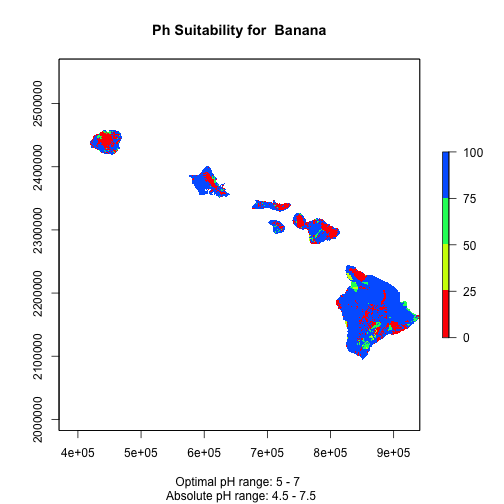``````# cleanup
rm(PH)
``````

A primary difference between the two is that the python script performs multiple condtional operations (i.e., rastcon1 through rastcon5) and output operations (i.e., true3 and true4) for each input value, before determining which conditional and output apply (see rast_suit, line 138). The R code reduces the total number of calculations by only determining outputs once a conditional is met (see soilsuit, lines 202-206). This truncating approach is taken for all scripts below.

Other than that the only differences are the that the python scripts pull in parameter data from a csv whereas currently only dummy data was selected and hardcorded in (e.g., ph_ABSmax etc).

# Depth Suitability

``````# Get variables
OPTmin <- locate("D_OPT")
ABSmin <- locate("D_ABS")

print(paste("Optimal minimum soil depth for", crop,"is:", OPTmin, "cm"))
``````
```##  "Optimal minimum soil depth for Banana is: 150 cm"
```
``````print(paste("Absolute minimum soil depth for", crop,"is:", ABSmin, "cm"))
``````
```##  "Absolute minimum soil depth for Banana is: 50 cm"
```
``````# define custom function
suit2min <- function(x) { ifelse(x < ABSmin, 0, # Conditional statement for values less than absolute minimum
ifelse(x >= OPTmin, 100, # Conditional for values greater than or equal to optimal minimum
ifelse(((x >= ABSmin) & (x < OPTmin)), ((x-ABSmin)/(OPTmin-ABSmin)*100), NA)))}

# run calculation
D_suit <- calc(DEPTH, suit2min)

# plot output
plot(D_suit, main= paste("Depth Suitability for ",crop), breaks = c(0,25,50,75,100), col = rainbow(5),
sub = paste('Optimal minimum depth is ', OPTmax, 'cm, \n absolute minimum depths is', ABSmax, 'cm.'))
``````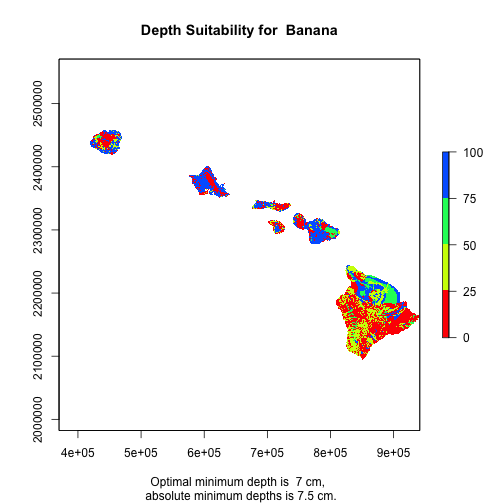# Slope Suitability

``````# Determine slope input variables
OPTmax <- as.integer(locate("S_OPTMAX"))
ABSmax <- as.integer(locate("S_ABSMAX"))

# Reivew texts
print(paste("Optimal maximum slope for", crop,"is:", OPTmax, "%"))
``````
```##  "Optimal maximum slope for Banana is: 28 %"
```
``````print(paste("Absolute maximum soil slope for", crop,"is:", ABSmax, "%"))
``````
```##  "Absolute maximum soil slope for Banana is: 38 %"
```
``````# Use fuzzy calculation to determine range of suitability for
suit2max <- function(x) { ifelse(x > ABSmax, 0, # Conditional statement for values greater than absolute maximum slope
ifelse(x <= OPTmax, 100, # Conditional statement for values less than or equal to optimal maximum slope
ifelse(((x <= ABSmax) & (x > OPTmax)),((1-(x - OPTmax)/(ABSmax-OPTmax))*100), NA)))} # Conditional statement for values greater than or equal absolute minimun AND less than optimal minimum

# calculate suitability
S_suit <- calc(SLOPE, suit2max)

plot(S_suit, main= paste("Slope Suitability for ",crop), breaks = c(0,25,50,75,100), col = rainbow(5),
sub = paste('Optimal maximum slope is ', OPTmax, '%, \n absolute maximum slope is', ABSmax, '%.'))
``````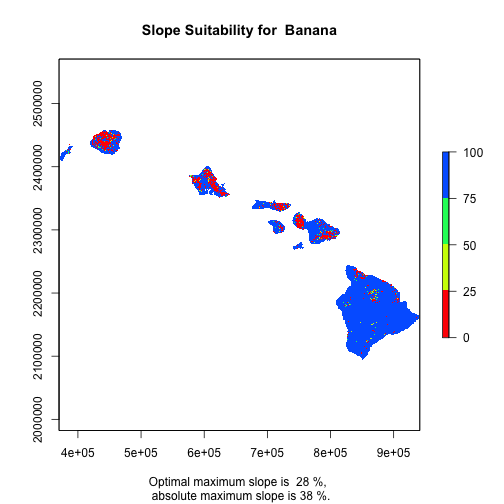# Drainage Suitability

``````# get variables
OPTmin <- as.numeric(locate("W_OPTMIN"))
OPTmax <- as.numeric(locate("W_OPTMAX"))
ABSmin <- as.numeric(locate("W_ABSMIN"))
ABSmax <- as.numeric(locate("W_ABSMAX"))

print(paste("Optimal soil drainage code range for", crop,"is:", OPTmin,"-",OPTmax))
``````
```##  "Optimal soil drainage code range for Banana is: 5.5 - 6.9"
```
``````print(paste("Absolute soil drainage code range for", crop,"is:", ABSmin,"-",ABSmax))
``````
```##  "Absolute soil drainage code range for Banana is: 2.9 - 9.1"
```
``````# calculate suitability
W_suit <- calc(DRAIN, suit4)

plot(W_suit, main= paste("Drainage Suitability for ",crop), breaks = c(0,25,50,75,100), col = rainbow(5),
sub=paste("Optimal drainage range:", OPTmin,"-",OPTmax, "\n Absolute Temperature range:", ABSmin,"-",ABSmax))
``````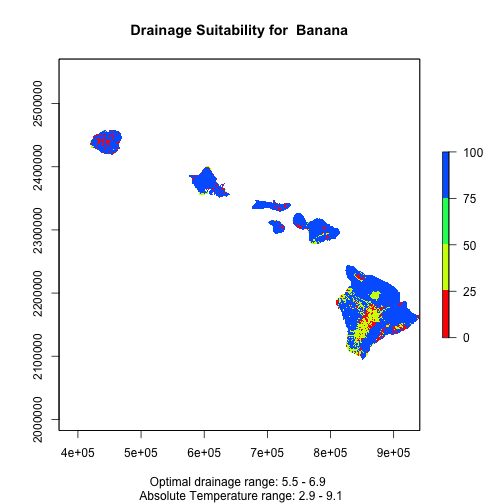### Calculate Temperature

``````# Get temp rasters
Env_Tmax <- Sys.glob("inputs/tmax/*.tif") %>% # get file list
sapply(raster) %>% #read in as rasters
stack() # store as raster stack

Env_Tmin <- stack(sapply(Sys.glob("inputs/tmin/*.tif"), raster)) # do same as above in one line

# Get float values for temperature variables from ecocrop db
ABSmax <- locate("T_ABSMAX")
ABSmin <- locate("T_ABSMIN")
OPTmax <- locate("T_OPTMAX")
OPTmin <- locate("T_OPTMIN")

print(paste("Optimal Temperature range for", crop,"is:", OPTmin,"-",OPTmax, "°C"))
``````
```##  "Optimal Temperature range for Banana is: 22 - 32 °C"
```
``````print(paste("Absolute Temperature range for", crop,"is:", ABSmin,"-",ABSmax, "°C"))
``````
```##  "Absolute Temperature range for Banana is: 13 - 38 °C"
```
``````## Suitability Evaluation
Tmax <- calc(Env_Tmax, suit4)
Tmin <- calc(Env_Tmin, suit4)

# make a raster stack with minimum value from min and max suitability layers by month

f <- function(x1, x2)  ifelse(x1 < x2, x1, x2)
T_suit <- overlay(Tmax, Tmin, fun=f, forcefun=TRUE)

#plot(T_suit, main= paste0("Temperature Suitability for ", crop), breaks = c(0,25,50,75,100), col = rainbow(5))

library(rasterVis)

levelplot(T_suit, main= paste0("Temperature Suitability for ", crop),
names.attr=month.name[1:12],
sub=paste("Optimal Temperature range:", OPTmin,"-",OPTmax, "°C \n Absolute Temperature range:", ABSmin,"-",ABSmax, "°C"),
at = seq(0, 100, by = 20),
col.regions = rainbow(5))
``````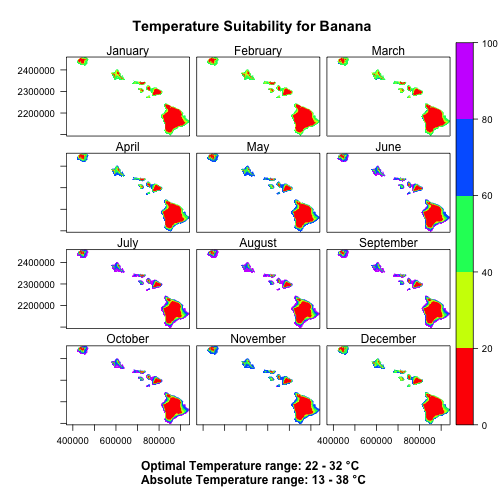Rainfall suitability analysis

``````# get list of Tif filenames and make raster stack
Env_rain <- sapply(Sys.glob("inputs/rainfall/*.tif"), raster) %>% stack()

# get variables
ABSmax <- locate("R_ABSMAX")/12
ABSmin <- locate("R_ABSMIN")/12
OPTmax <- locate("R_OPTMAX")/12
OPTmin <- locate("R_OPTMIN")/12

# Get float values for precipitation variables from ecocrop csv file:
print(paste("Optimal Precipitation range for", crop,"is:", OPTmin,"-",OPTmax, "mm"))
``````
```##  "Optimal Precipitation range for Banana is: 200 - 225 mm"
```
``````print(paste("Absolute Precipitation range for", crop,"is:", ABSmin,"-",ABSmax, "mm"))
``````
```##  "Absolute Precipitation range for Banana is: 166.666666666667 - 291.666666666667 mm"
```
``````# calculate suitability
R_suit <- calc(Env_rain, suit4)

# plot outputs
levelplot(R_suit, main= paste0("Precipitation Suitability for ", crop),
names.attr=month.name[1:12],
sub=paste("Optimal Precipitation range:", round(OPTmin),"-",round(OPTmax), "mm \n Absolute Precipitation range:", round(ABSmin),"-",round(ABSmax), "mm"),
at = seq(0, 100, by = 20),
col.regions = rainbow(5))
``````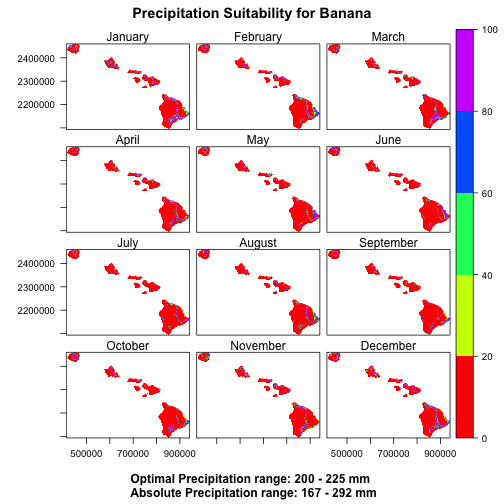### Seasonal Calculations

``````# Get the min and max days of season,
# divide and round up to produce integer for season lenght in months

#Cmin <- as.integer(ceiling(locate("C_MIN")/30.42))
Cmax <- as.integer(ceiling(locate("C_MAX")/30.42)) # 365/12=30.42

print(paste("Maximum growing season length for", crop,"is:", Cmax, "months"))
``````
```##  "Maximum growing season length for Banana is: 12 months"
```
``````#MAKE ONE GIANT SEASON ASSESSMENT FUNCTION
season_avg <- function(var){
# make double stack of monthly input variable (R or T)
months24 <- stack(var, var)
# months24 <- stack(R_suit, R_suit)

# Make a matrix of all growing season possibiliites:
# index season by col
seasons <- sapply(1:12, function(n) c(n, n+Cmax-1))

# make a list of stack
z <- sapply(1:12, function(i) months24[[seasons[1,i]:seasons[2,i]]])

# if Cmax >= 12, run first season stack mean, else run all seasons

# seasons_mean <- if (Cmax >= 12) calc(z[], mean)
# seasons_mean <- if (Cmax < 12) sapply(1:2, function(i) calc(z[i][], mean))
#
agg.fun <- function(x,...) { ## you should add ... for na.rm,and other extra parameters

if (Cmax < 12)return(calc(x[], mean)) # REPLACE wih Cmax >= 12
else{
return(stack(sapply(1:2, function(i) calc(z[i][], mean))))  # REPLACE wih sapply(1:12,... and remove stack?
}
}

seasons_mean <- agg.fun(z)
return(seasons_mean)
}

#Check if RAINFALL is annual or monthly
Rcat <- if (dim(R_suit) == 12) season_avg(R_suit)
Tcat <- if (dim(T_suit) == 12) season_avg(T_suit)

# Rcat <- Rcat1
# Tcat <- Tcat1

################### RESAMPLE STEP NEEDS TO BE REMOVED AND FIXED EARLIER
Rcat <- resample(Rcat,Tcat,method='ngb')

#Create Rain by Temp suitability
rainxtemp <- function(x,y){
rast_stack <- stack(x,y)
fun <- function(x) { ((x[] * x[]) / 100)}
RxT <- calc(rast_stack, fun)
return(RxT)
}

#if TEMP & RAIN is monthly, then run the rainxtemp function defined above
RxxT <- if((dim(R_suit) == 12) & (dim(T_suit) == 12)) rainxtemp(Rcat, Tcat)

plot(RxxT)
``````